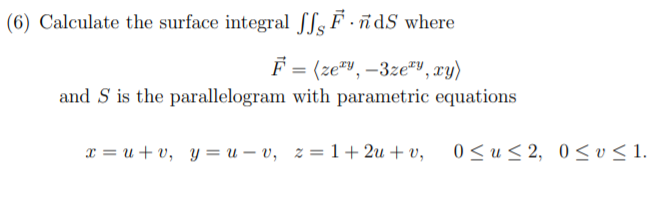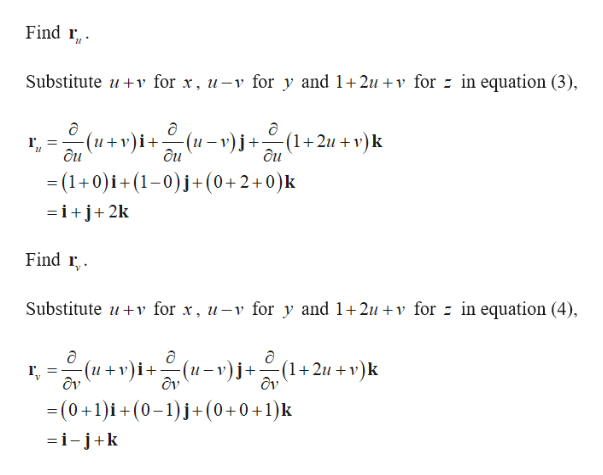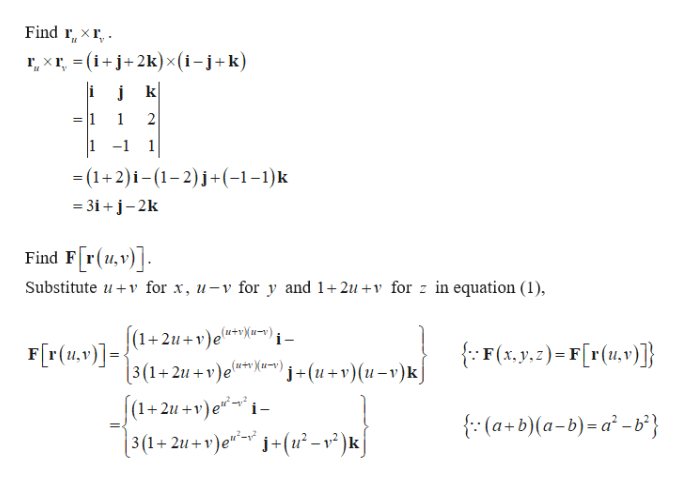# (6) Calculate the surface integral SSs F · ñdS whereF = (ze", –3ze"V, xy)and S is the parallelogram with parametric equations0 < u < 2,0< v < 1.x = u + v, y = u – v, z = 1+ 2u + v,

Question
136 viewshelp_outlineImage Transcriptionclose(6) Calculate the surface integral SSs F · ñdS where F = (ze", –3ze"V, xy) and S is the parallelogram with parametric equations 0 < u < 2, 0< v < 1. x = u + v, y = u – v, z = 1+ 2u + v, fullscreen
check_circle

Step 1

Given that,

Step 2help_outlineImage TranscriptioncloseFind r, . Substitute u+v for x, u-v for y and 1+2u +v for = in equation (3), -(u – v) j+- (1+2u+v)k ôu -(u+v)i+ ôu ôu = (1+0)i+(1-0)j+(0+2+0)k =i+j+2k Find r, . Substitute u+v for x, u-v for y and 1+2u +v for - in equation (4), -(u + v)i+(u-v)j+÷(1+2u+v)k dv = (0+1)i+(0-1)j+(0+0+1)k =i-j+k fullscreen
Step 3help_outlineImage TranscriptioncloseFind r„ × r, - r, × r, = (i+j+2k)×(i-j+k) 2 1 -1 = (1+2)i– (1–2)j+(-1-1)k = 3i + j-2k Find F[r(4,v)]. Substitute u+v for x, u-v for y and 1+2u +v for z in equation (1), F[r(u,v)]={(1+2u+v)e*»Ye~)} - [3(1+2u + v)e' F[r(u.v)]= {: F(x,y.2) = F[r(1u,v)]} ™*X* j+(u+v)(u-v)k] (1+2u +v)e™~* i – {: (a+b)(a-b)=a² -b³} 3(1+ 2u + v)e*- j+(u² – v² )k] fullscreen

### Want to see the full answer?

See Solution

#### Want to see this answer and more?

Solutions are written by subject experts who are available 24/7. Questions are typically answered within 1 hour.*

See Solution
*Response times may vary by subject and question.
Tagged in

### Integration